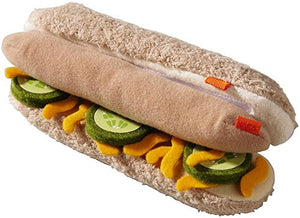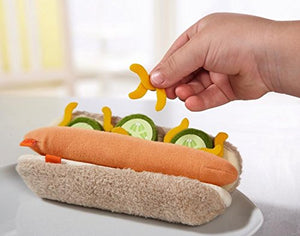# Haba Biofino Hot Dog With Bun And Toppings

Vendor
HABA
Regular price
\$42.54
Sale price
\$42.54
Quantity must be 1 or more

• T

• h

• i

• s

• p

• l

• u

• s

• h

• h

• o

• t

• d

• o

• g

• c

• o

• m

• e

• s

• w

• i

• t

• h

• l

• o

• t

• s

• o

• f

• f

• u

• n

• t

• o

• p

• p

• i

• n

• g

• s

• !

• T

• h

• e

• p

• o

• l

• y

• e

• s

• t

• e

• r

• a

• n

• d

• f

• e

• l

• t

• s

• e

• t

• i

• n

• c

• l

• u

• d

• e

• s

• 2

• h

• a

• l

• v

• e

• s

• o

• f

• a

• b

• a

• k

• i

• n

• g

• r

• o

• l

• l

• ,

• 1

• h

• o

• t

• d

• o

• g

• ,

• 3

• s

• l

• i

• c

• e

• s

• o

• f

• c

• u

• c

• u

• m

• b

• e

• r

• ,

• a

• n

• d

• s

• e

• v

• e

• r

• a

• l

• c

• h

• e

• e

• s

• e

• s

• h

• r

• e

• d

• s

• /

• r

• o

• a

• s

• t

• e

• d

• o

• n

• i

• o

• n

• s

• .

Cook up the fun with the HABA Hot Dog! Ideal for all kinds of pretend play, play food from HABA is highly detailed and always charmingly designed. This plush hot dog comes with lots of fun toppings! The polyester and felt set includes 2 halves of a baking roll, 1 hot dog, 3 slices of cucumber, and several cheese shreds/roasted onions. This cute set is an excellent addition to any play kitchen or grocery store. Perfect for ages 3 years and up.